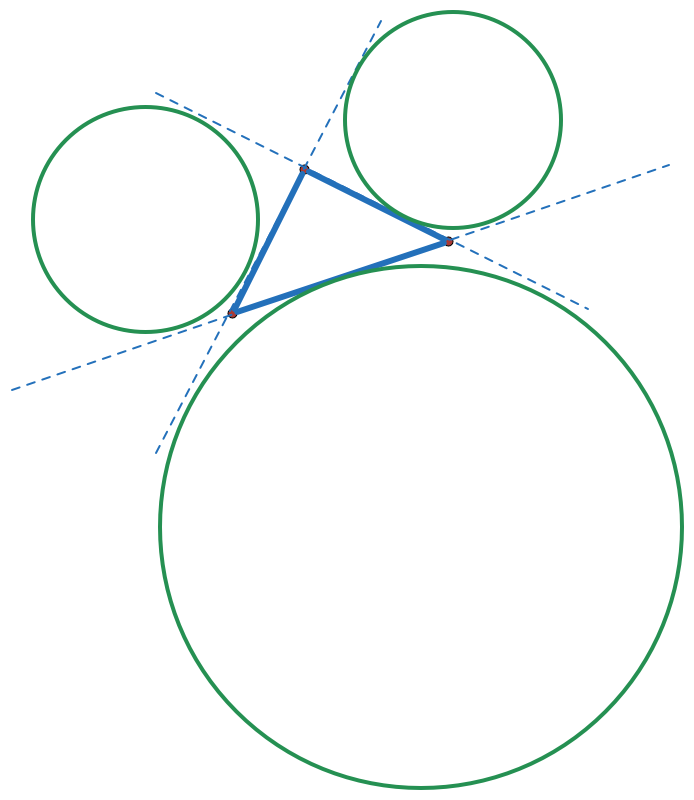Mickey Mouse?If $a,b,$ and $c$ are the side lengths of $\triangle ABC$ opposite to angles $A,B,$ and $C,$ respectively, and $r_{1},r_{2},$ and $r_{3}$ are the corresponding exradii, then find the value of

$\frac {b-c}{r_{1}} + \frac {c-a}{r_{2}} + \frac{a-b}{r_{3}}.$

×